• 下面小编就为大家分享一篇使用pandas中的DataFrame数据绘制柱状图的方法，具有很好的参考价值，希望对大家有所帮助。一起跟随小编过来看看吧
• 封装接口直接利用DataFrame绘制百分比柱状图1. 背景前言2. 官方网址示例2.1三级目录 手动反爬虫： 知识梳理不易，请尊重劳动成果，文章仅发布在CSDN网站上，在其他网站看到该博文均属于未经作者授权的恶意爬取信息 ...
封装接口直接利用DataFrame绘制百分比柱状图1. 背景前言2. 官方网址示例2.1 matplotlib_percentage_stacked_bar_plot2.2 percent-stacked-barplot2.3 Discrete distribution as horizontal bar chart3. 问题解决3.1 全部代码3.2 代码试错4. 实操检验4.1 实操测试4.2 更换标签4.3 部分标签输出
手动反爬虫：原博地址 https://editor.csdn.net/md/?articleId=106524459
 知识梳理不易，请尊重劳动成果，文章仅发布在CSDN网站上，在其他网站看到该博文均属于未经作者授权的恶意爬取信息

本文为付费内容，禁止转载
1. 背景前言
最近打比赛遇到的问题有点多，在绘制了堆叠柱状图之后，队长说不仅要看到具体的数量多少的堆叠图，还要看到具体占比的百分比柱状图，具体的样例可参考灵魂画图，因此也就产生了绘制百分比柱状图的需求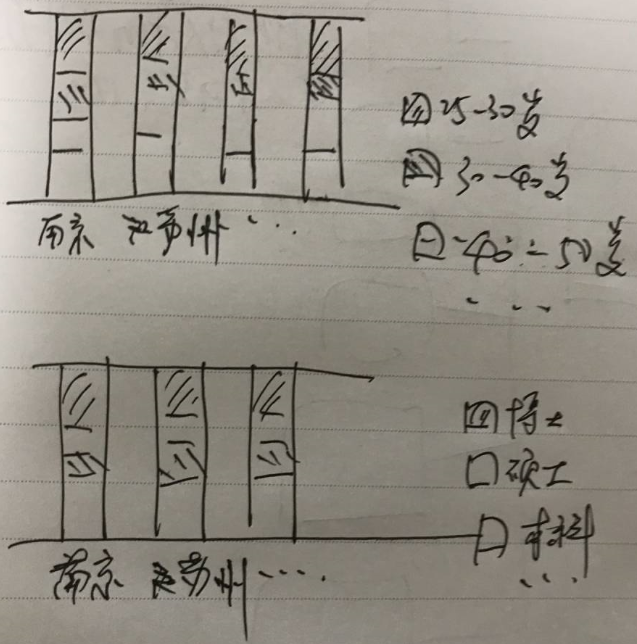2. 官方网址示例
2.1 matplotlib_percentage_stacked_bar_plot
示例网址1
里面的代码是真的长，完全属于一步步进行“堆砖块”然后才形成的百分比堆叠图，最终得出图结果如下：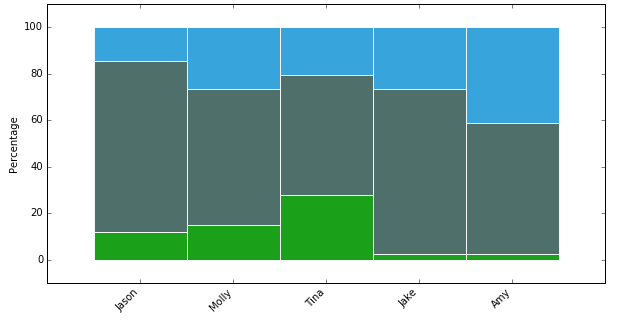2.2 percent-stacked-barplot
示例网址2
这个网址给出的代码比第一个网址给出的较为简洁，但是本质上都是属于一个个“砖块”的叠加，代码及生成的示例图形如下：
# libraries
import numpy as np
import matplotlib.pyplot as plt
from matplotlib import rc
import pandas as pd

# Data
r = [0,1,2,3,4]
raw_data = {'greenBars': [20, 1.5, 7, 10, 5], 'orangeBars': [5, 15, 5, 10, 15],'blueBars': [2, 15, 18, 5, 10]}
df = pd.DataFrame(raw_data)

# From raw value to percentage
totals = [i+j+k for i,j,k in zip(df['greenBars'], df['orangeBars'], df['blueBars'])]
greenBars = [i / j * 100 for i,j in zip(df['greenBars'], totals)]
orangeBars = [i / j * 100 for i,j in zip(df['orangeBars'], totals)]
blueBars = [i / j * 100 for i,j in zip(df['blueBars'], totals)]

# plot
barWidth = 0.85
names = ('A','B','C','D','E')
# Create green Bars
plt.bar(r, greenBars, color='#b5ffb9', edgecolor='white', width=barWidth)
# Create orange Bars
plt.bar(r, orangeBars, bottom=greenBars, color='#f9bc86', edgecolor='white', width=barWidth)
# Create blue Bars
plt.bar(r, blueBars, bottom=[i+j for i,j in zip(greenBars, orangeBars)], color='#a3acff', edgecolor='white', width=barWidth)

# Custom x axis
plt.xticks(r, names)
plt.xlabel("group")

# Show graphic
plt.show()

→ 输出的结果为：（这个结果相对好一点）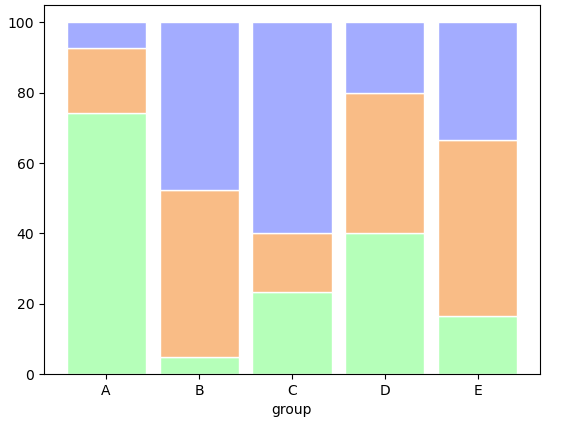2.3 Discrete distribution as horizontal bar chart
示例网址3
官方给出的示例图是下面这样的，看的第一眼是非常符合自己的要求的，而且还有不同的配色以及主题的标签设置及文本文字标注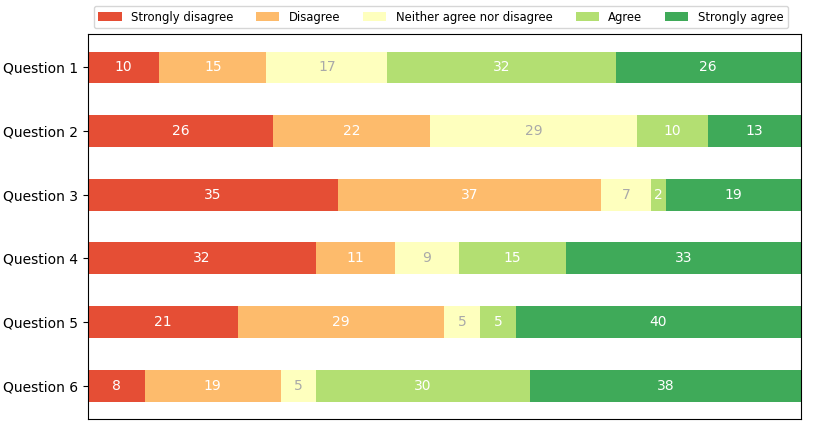接着就看一下给出的源码，如下，代码封装的挺不错的，还有可以直接调用的函数，按照官方示例的代码运行后的确可以实现上方的图形效果（内心狂喜~~ ）
import numpy as np
import matplotlib.pyplot as plt

category_names = ['Strongly disagree', 'Disagree',
'Neither agree nor disagree', 'Agree', 'Strongly agree']
results = {
'Question 1': [10, 15, 17, 32, 26],
'Question 2': [26, 22, 29, 10, 13],
'Question 3': [35, 37, 7, 2, 19],
'Question 4': [32, 11, 9, 15, 33],
'Question 5': [21, 29, 5, 5, 40],
'Question 6': [8, 19, 5, 30, 38]
}

def survey(results, category_names):
"""
Parameters
----------
results : dict
A mapping from question labels to a list of answers per category.
It is assumed all lists contain the same number of entries and that
it matches the length of *category_names*.
category_names : list of str
The category labels.
"""
labels = list(results.keys())
data = np.array(list(results.values()))
data_cum = data.cumsum(axis=1)
category_colors = plt.get_cmap('RdYlGn')(
np.linspace(0.15, 0.85, data.shape))

fig, ax = plt.subplots(figsize=(9.2, 5))
ax.invert_yaxis()
ax.xaxis.set_visible(False)
ax.set_xlim(0, np.sum(data, axis=1).max())

for i, (colname, color) in enumerate(zip(category_names, category_colors)):
widths = data[:, i]
starts = data_cum[:, i] - widths
ax.barh(labels, widths, left=starts, height=0.5,
label=colname, color=color)
xcenters = starts + widths / 2

r, g, b, _ = color
text_color = 'white' if r * g * b < 0.5 else 'darkgrey'
for y, (x, c) in enumerate(zip(xcenters, widths)):
ax.text(x, y, str(int(c)), ha='center', va='center',
color=text_color)
ax.legend(ncol=len(category_names), bbox_to_anchor=(0, 1),
loc='lower left', fontsize='small')

return fig, ax

survey(results, category_names)
plt.show()

这个官方给出的代码，看似可以解决问题，但是直接调用之后发现，并不是百分比柱状图的结果，比如这里将数据稍作修改，更改数据如下，然后再运行代码
results = {
'Question 1': [10, 15, 17, 32, 26],
'Question 2': [26, 22, 29, 10, 13],
'Question 3': [35, 37, 7, 2, 19],
'Question 4': [32, 11, 9, 15, 33],
'Question 5': [21, 29, 5, 5, 40],
'Question 6': [8, 190, 5, 30, 38] #在19后面加个0
}

→ 输出的结果为：（实际上这个图本身就不是百分比堆叠柱状图，根据官方的名称是离散分布的水平柱形图）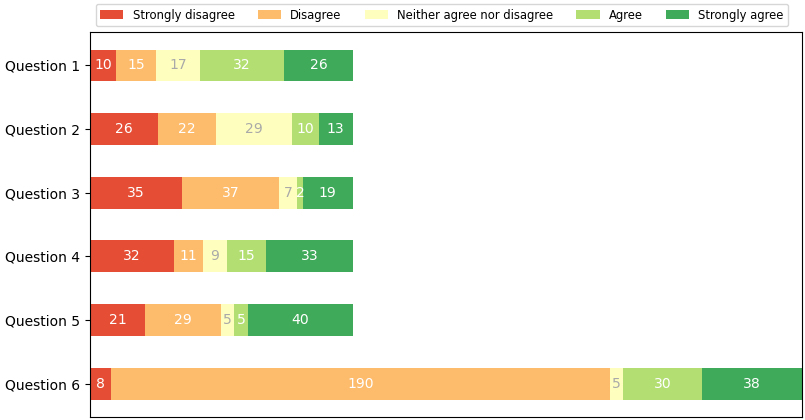然后百度搜索相关的python绘制的百分比堆叠柱状图基本上都是进行一个个“垒砌”的，看到百度搜索返回的第一个结果，竟然把这个官方的示例声称为自己封装的函数，标题还是绘制百分比柱状图，误导读者（本人也是被忽悠了，直到看到官网示例和实际调试）
3. 问题解决
既然没有现成的可以直接copy的代码，而且也没有像绘制堆叠图时候直接就拥有stacked的参数，所以就必须自己手动整理了。前面的第三个示例代码的框架可以直接拿过来用，就不用再进行编写了，只需要修改部分内容即可
3.1 全部代码
仿照之前的代码形式，进行代码改写，只需要传入DataFrame数据即可，如下
def percentage_bar(df):
labels = df.index.tolist() #提取分类显示标签
results = df.to_dict(orient = 'list') #将数值结果转化为字典
category_names = list(results.keys()) # 提取字典里面的类别（键-key）
data = np.array(list(results.values())) #提取字典里面的数值（值-value）

category_colors = plt.get_cmap('RdYlGn')(np.linspace(0.15, 0.85, data.shape))
#设置占比显示的颜色，可以自定义，修改括号里面的参数即可，如下
#category_colors = plt.get_cmap('hot')(np.linspace(0.15, 0.85, data.shape))

fig, ax = plt.subplots(figsize=(12, 9)) #创建画布，开始绘图
ax.invert_xaxis()#这个可以通过设置df中columns的顺序调整
ax.yaxis.set_visible(False) #设置y轴刻度不可见
ax.set_xticklabels(labels=labels, rotation=90) #显示x轴标签，并旋转90度
ax.set_ylim(0,1) #设置y轴的显示范围
starts = 0  #绘制基准
for i, (colname, color) in enumerate(zip(category_names, category_colors)):
heights = data[i,: ]/ data.sum(axis =0) #计算出每次遍历时候的百分比
ax.bar(labels, heights, bottom=starts, width=0.5,label=colname, color=color,edgecolor ='gray') # 绘制柱状图
xcenters = starts + heights/2  #进行文本标记位置的选定
starts += heights  #核心一步，就是基于基准上的百分比累加
#print(starts) 这个变量就是能否百分比显示的关键，可以打印输出看一下
percentage_text = data[i,: ]/ data.sum(axis =0) #文本标记的数据

r, g, b, _ = color  # 这里进行像素的分割
text_color = 'white' if r * g * b < 0.5 else 'k'  #根据颜色基调分配文本标记的颜色
for y, (x, c) in enumerate(zip(xcenters, percentage_text)):
ax.text(y, x, f'{round(c*100,2)}%', ha='center', va='center',
color=text_color, rotation = 90) #添加文本标记
ax.legend(ncol=len(category_names), bbox_to_anchor=(0, 1),
loc='lower left', fontsize='large') #设置图例
return fig, ax  #返回图像

比如把刚刚示例中的数据转化为DataFrame数据，如下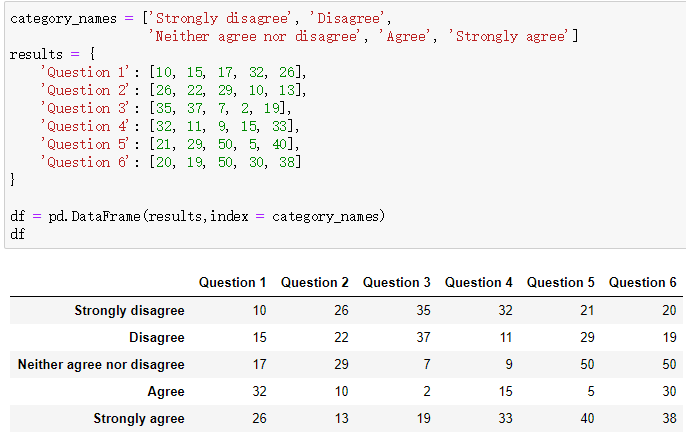然后将df作为参数直接传递到percentage_bar函数中，看看结果输出，内部虽然设置x轴标签旋转90度，但是在函数外边还是可以直接调整其方向，这里就是取消旋转了，而且在最后的文本标记中采用的是百分比进行进标注的，这样更能清晰的明确各方的占比情况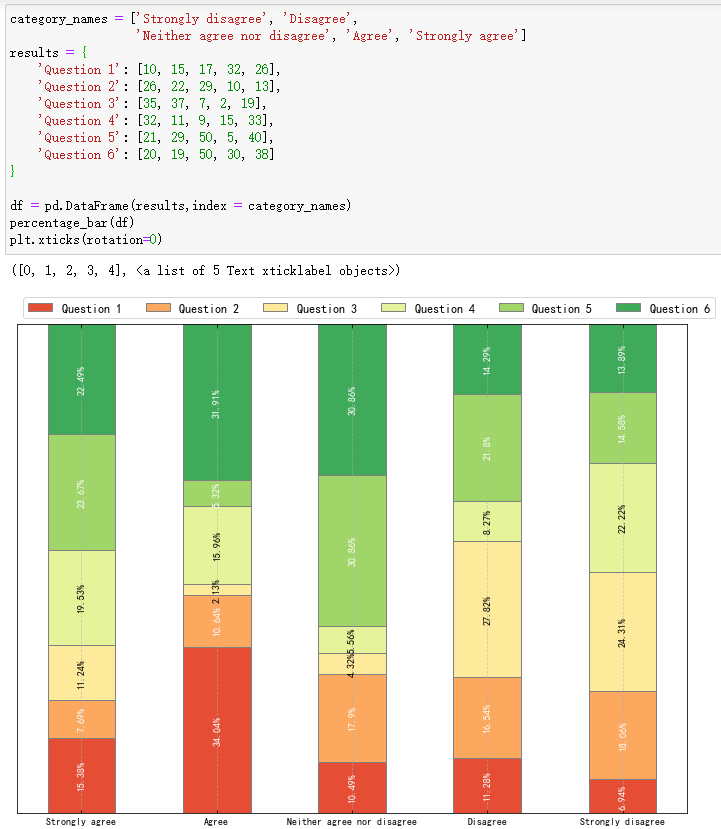3.2 代码试错
还是和最初一样，在最后一行数据的19后面添加一个0，变成190，这里顺带将y轴的刻度范围调整为（0,1.1），来查看是否符合百分比的要求，然后执行代码及输出结果如下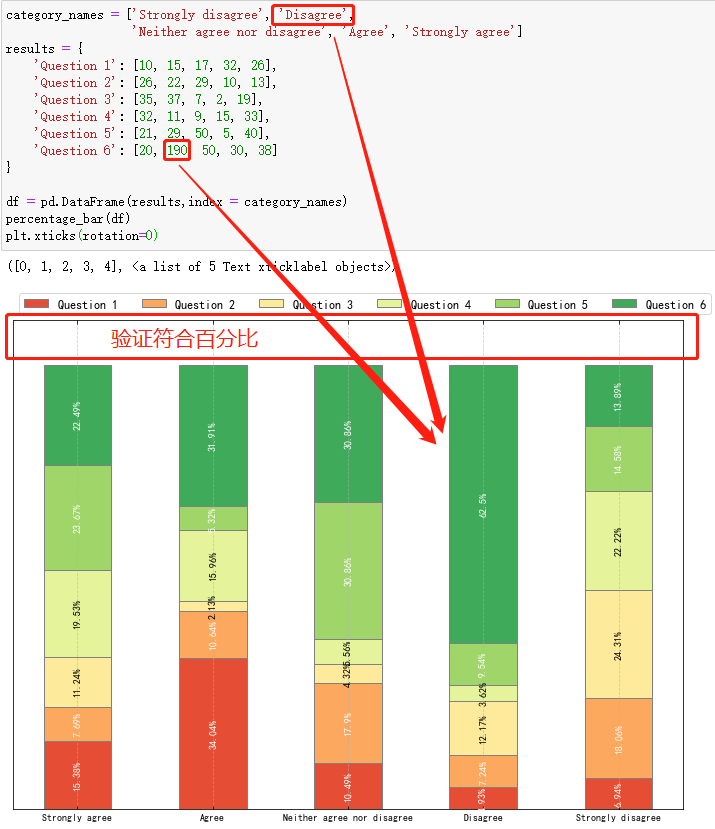4. 实操检验
上面已经对函数进行了试错，无误后直接将函数放置到自己自定义的第三方模块下，方便自己调用，关于这步的操作，可以参考博客将自定义常用的一些函数封装成可以直接调用的模块方法
4.1 实操测试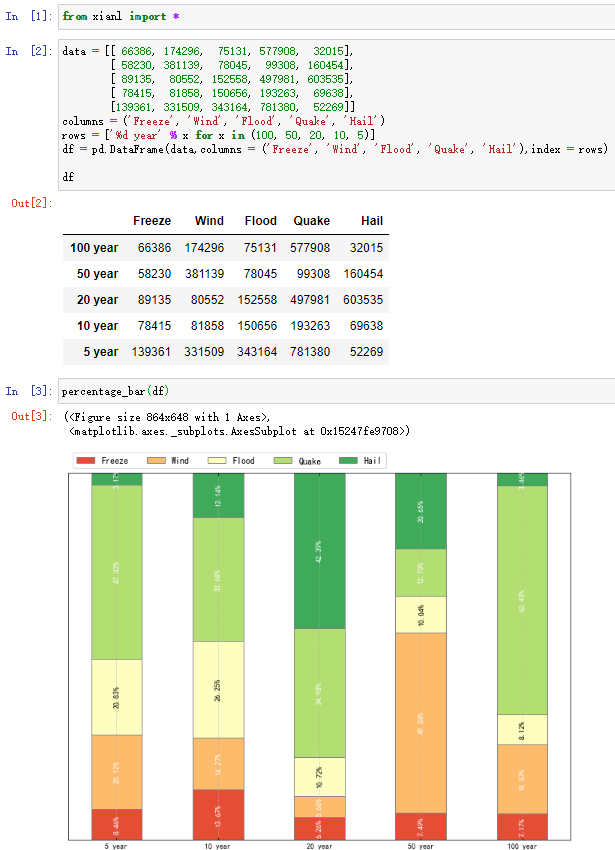4.2 更换标签
如果需要更换标签显示（df的标签与列标题互换），只需要将df转置即可，如下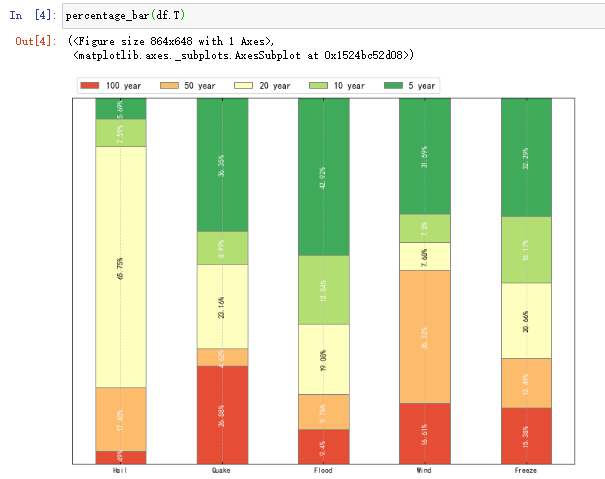4.3 部分标签输出
如果需要输出部分的标签，可以直接对df的columns进行切片即可（如果更换标签index转置之后也是同理），输出如下，比如这里只取两个标签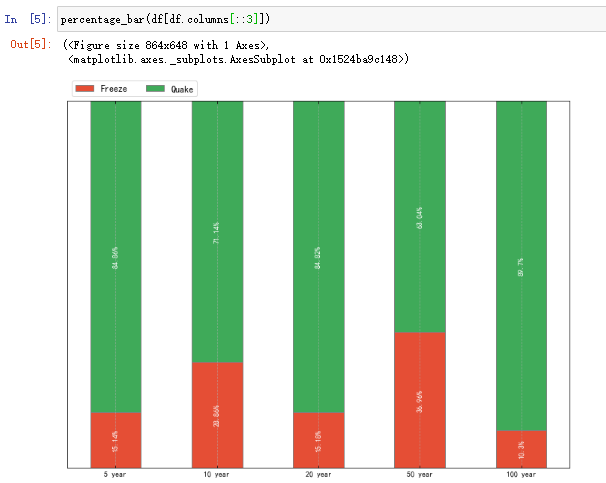最后，梳理不易，码字分享希望能够帮助大家。


展开全文python 数据可视化 数据分析 matplotlib
• 使用pandas的DataFrame方法进行柱状图绘制也是比较方便的。 把之前的折线图绘制代码修改一下如下：  1 from pandas import Series,DataFrame  2 from numpy.random import randn  3 import numpy as
折线图是数据分析的一种手段，但是有时候我们也需要柱状图进行不同数据的可视化量化对比。使用pandas的DataFrame方法进行柱状图的绘制也是比较方便的。
把之前的折线图绘制代码修改一下如下：
1 from pandas
import Series,DataFrame
2 from numpy.random
import randn
3 import numpy
as np
4 import matplotlib.pyplot
as plt
5
6 df = DataFrame(abs(randn(10,5)),columns=['A','B','C','D','E'],index
= np.arange(0,100,10))
7 df.plot(kind='bar')
8 plt.show()
9

程序执行结果如下：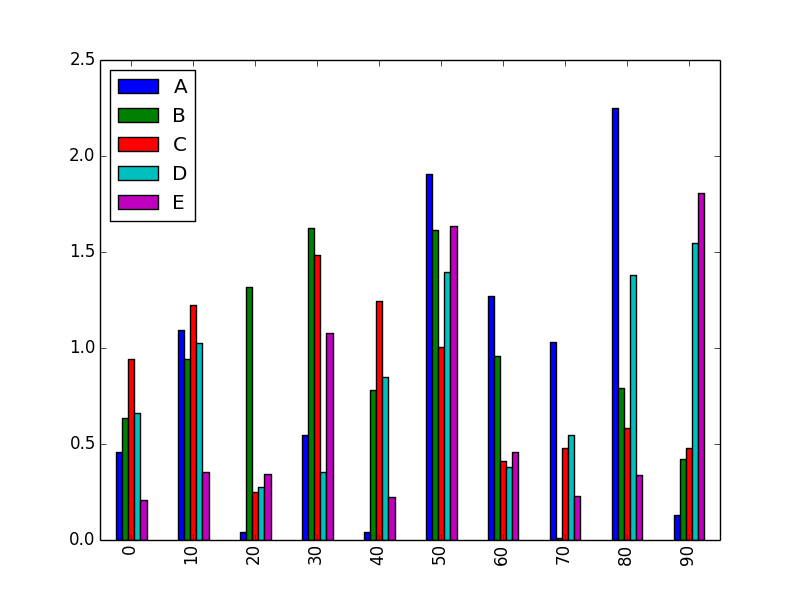这种绘制方式确实是让人很欣喜，我觉得在一定程度上比Excel的数据处理要便捷很多。对于我这种对Excel一点都不熟悉的我来说，这种方法已经好多了。

展开全文• 使用pandas的DataFrame方法进行柱状图绘制也是比较方便的。把之前的折线图绘制代码修改一下如下：from pandas import Series,DataFramefrom numpy.random import randnimport numpy as npimport matplotlib.pyp...
折线图是数据分析的一种手段，但是有时候我们也需要柱状图进行不同数据的可视化量化对比。使用pandas的DataFrame方法进行柱状图的绘制也是比较方便的。把之前的折线图绘制代码修改一下如下：from pandas import Series,DataFramefrom numpy.random import randnimport numpy as npimport matplotlib.pyplot as pltdf = DataFrame(abs(randn(10,5)),columns=['A','B','C','D','E'],index = np.arange(0,100,10))df.plot(kind='bar')plt.show()程序执行结果如下：这种绘制方式确实是让人很欣喜，我觉得在一定程度上比Excel的数据处理要便捷很多。对于我这种对Excel一点都不熟悉的我来说，这种方法已经好多了。以上这篇使用pandas中的DataFrame数据绘制柱状图的方法就是小编分享给大家的全部内容了，希望能给大家一个参考，也希望大家多多支持我们。本文标题: 使用pandas中的DataFrame数据绘制柱状图的方法本文地址: http://www.cppcns.com/jiaoben/python/224897.html
展开全文• import pandas as pd import numpy as np import matplotlib.pyplot as plt from pandas import Series ...#Series画柱状图 fig,axes=plt.subplots(2,1)#两行一列 data=Series(np.random.rand(16)...
import pandas as pd
import numpy as np
import matplotlib.pyplot as plt
from pandas import Series
from pandas import DataFrame
#Series画柱状图
fig,axes=plt.subplots(2,1)#两行一列
data=Series(np.random.rand(16),index=list("abcdefghijklmnop"))
print(data)
data.plot(kind="bar",ax=axes,color="k",alpha=0.7)#垂直柱状图,ax是要在其上进行绘制的subplot的对象
data.plot(kind="barh",ax=axes,color="k",alpha=0.7)#水平柱状图
plt.show()
#DataFrame画柱状图
df=DataFrame(np.random.rand(6,4),columns=pd.Index(["A","B","C","D"],name="Genus"),index=pd.Index(["one","two","three","four","five","six"],name="age"))
print(df)
df.plot(kind="barh",stacked=True,alpha=0.5)
#df.plot(kind="bar")#垂直柱状图
#df.plot(kind="bar",stacked="True")#生成堆积柱状图
plt.show()
展开全文• 在pandas dataframe中使用plot画图，当x轴是日期的时候，x轴会显示时间和分钟。如果使用set_major_formatter函数格式化ax.xaxis.set_major_formatter(DateFormatter('%Y-%m-%d'));那么会报错ValueError: ...
• 目录1 问题2 从柱状图绘制完整统计图注意事项3 Python3代码4 参考文献1 问题同样是统计图，有的美观的表达了想法，有的却让人看不明白。那么，我们在绘制完整统计图时要注意哪些事项呢？下面通过柱状图来说明。2 ...
• 之前写了个服务器磁盘监控的脚本，每天将磁盘的使用情况通过电子邮件发送出来，通过paramiko.SSHClient()将磁盘使用情况报错到一个DataFrame对象中，然后通过DataFrame的实例方法...所以想改造成直接显示柱状图。但是邮
• 柱状图大家都会制作，那么你会用python制作柱状图吗？我们先来看一下柱状图的定义：柱形图，又被称为长条图、或者柱状统计图，也叫作条图、条状图、棒形图，本质上来说就是一种以长方形的长度为变量的统计图表。长条...
• height 表示柱状图的高度，也就是y坐标值，数据类型为int或float类型， width 表示柱状图的宽度，取值在0~1之间，默认为0.8 bottom 柱状图的起始位置，也就是y轴的起始坐标， align 柱状图的中心位置，"center","le...python 详解
• 在使用pandas进行绘图时，发现运行如下代码没有显示结果： ...dataframe.plot(kind='bar') 在经过一番尝试之后，发现修改为如下代码即可显示： plt.figure() dataframe.plot(kind='bar') plt.show() ...Pandas
• 柱状图是一种可以简单的比对数据间差异的一种统计图，在日常的数据分析中应用是相当广泛的，在python中也可以使用matplotlib绘制柱状图，不过很多小伙伴在使用matplotlib的时候会觉得matplotlib柱状图参数的设置有点...
• ‘hist’ : histogram#柱状图 ‘box’ : boxplot#箱线图 ‘kde’ : Kernel Density Estimation plot#Kernel 的密度估计图，主要对柱状图添加Kernel 概率密度线 ‘density’ : same as ‘kde’ ‘area’ : area plot#...
• import pandas as pd import matplotlib . pyplot as ...# 柱状图 ...# 横向柱状图 ...累计柱状图： ...# 累计柱状图 ...DataFrame ...# histtype='stepfilled'柱状图之间没有间隔 bins=30，直方图的宽度Pandas
• 1.柱形。import matplotlib.pylab as pltimport seaborn as snsimport pandas as pddf = pd.DataFrame()df['group'] = ['A','B','C']df['value'] = [12, 4, 8]p1=sns.barplot( data=df, x='group', y='value' )plt...
• 柱状图，英文名: column chart, 或者bar chart。柱状图又称为条形图，属于比较类；其描述的是分类数据；以可视化的方式展示有多少分类；不能显示趋势化类的数据工具/原料Anaconda3.exe方法/步骤1首先，我们使用...
• 问题描述：在当前文件夹中有一个存放同一门课程两个班级同学成绩的Excel文件“学生成绩.xlsx”，每个工作表中存放一个班级的成绩。编写程序，使用pandas读取其中的数据，然后绘制柱状图...数据分析 数据可视化 可视化 编程语言 excel
• 我们经常看到的Bar Chart Race（柱形竞赛），可以看到数据的呈现非常的直观。今天就一起来学习下如何生成和上面一样的柱形竞赛。1、导入Python库Python12345importpandasaspdimportmatplotlib....
• 在python中，为什么画柱状图，顺序没有按照给出的列表来有可能是最大值和最小值之间差别太大了，按比例最小值无法显示了。试试把值y改成log(y)显示呢？那样也许会平缓点。pythonK线的柱状图怎么画里提了一个自问自...
• 成功解决采用ax.bar进行三维绘图绘制柱状图的时候，横坐标只显示三列而不是数据中的四列 目录 解决问题 解决思路 解决方法 解决问题 输入数据为四列五行，如下所示： labelX_lists02= ['MAE_Default...
• 如果要将图形显示为分类条形，即独立于实际日期的等距条形，可以重新格式化xticklabelsf = lambda x: datetime.datetime.strptime(x, '%Y-%m-%d %H:%M:%S').strftime('%b')ax.set_xticklabels([ f(x.get_text())...
• import pandas as pd a = [1, 1, 2, 1, 1, 4, 5, 6] df = pd.DataFrame(a, columns=['a']) Second, the geom is geom_histogram() not geom_hist(). And finally, it looks like you're throwing in code from one ......